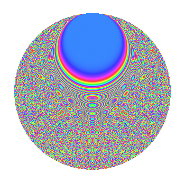# Properties

 Label 3234.2.beLevel 3234 Weight 2 Character orbit be Rep. character $$\chi_{3234}(659,\cdot)$$ Character field $$\Q(\zeta_{14})$$ Dimension 1344 Sturm bound 1344

# Related objects

## Defining parameters

 Level: $$N$$ $$=$$ $$3234 = 2 \cdot 3 \cdot 7^{2} \cdot 11$$ Weight: $$k$$ $$=$$ $$2$$ Character orbit: $$[\chi]$$ $$=$$ 3234.be (of order $$14$$ and degree $$6$$) Character conductor: $$\operatorname{cond}(\chi)$$ $$=$$ $$1617$$ Character field: $$\Q(\zeta_{14})$$ Sturm bound: $$1344$$

## Dimensions

The following table gives the dimensions of various subspaces of $$M_{2}(3234, [\chi])$$.

Total New Old
Modular forms 4080 1344 2736
Cusp forms 3984 1344 2640
Eisenstein series 96 0 96

## Trace form

 $$1344q - 224q^{4} + O(q^{10})$$ $$1344q - 224q^{4} - 224q^{16} - 10q^{22} + 216q^{25} + 60q^{27} + 8q^{31} - 16q^{33} - 8q^{34} - 8q^{37} + 88q^{42} + 20q^{45} - 72q^{49} - 10q^{55} - 160q^{58} - 224q^{64} + 8q^{66} - 16q^{67} - 60q^{70} + 104q^{75} - 32q^{78} + 104q^{81} - 8q^{82} + 4q^{88} + 200q^{91} + 40q^{93} + 32q^{97} + 200q^{99} + O(q^{100})$$

## Decomposition of $$S_{2}^{\mathrm{new}}(3234, [\chi])$$ into newform subspaces

The newforms in this space have not yet been added to the LMFDB.

## Decomposition of $$S_{2}^{\mathrm{old}}(3234, [\chi])$$ into lower level spaces

$$S_{2}^{\mathrm{old}}(3234, [\chi]) \cong$$ $$S_{2}^{\mathrm{new}}(1617, [\chi])$$$$^{\oplus 2}$$

## Hecke characteristic polynomials

There are no characteristic polynomials of Hecke operators in the database